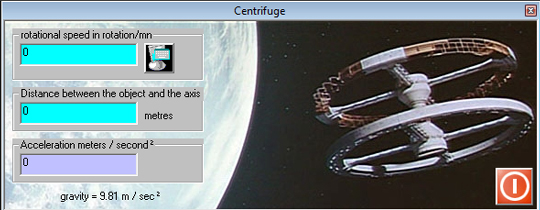The gravity plays a key role in the equations of fluid mechanics, the density of the fluid is gravity factor in the equation of the theorem Bernoulli

• Gravity or acceleration is measured in meters / second² )
• on the earth it is 9.81m/s² to the poles and 9.78m/s² at the equator (the effect of centrifugal force)
• on the moon that acceleration is 1.62m/s²• gravity acceleration g : Based on the assumption :"any force acting alone is proportional to the acceleration" and considering that " the weight of an object (the force applied thereto) is the force, gravity is determined as the acceleration of the mass of an object , which gives the weight of the object
Examples of the effects of gravity (accident, elevator ..) in the tab mass and acceleration ofthe converter included in Mecaflux.

Mecaflux standard is supplied with the converter that allows the various calculations in conjunction with acceleration..

mass acceleration deceleration relationship weight / shock in the converter

relation kinetic energy acceleration in the converter